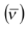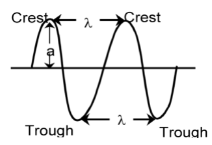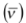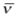# Some Important Characteristics of A Wave

## Atomic Structure of Class 11

A wave is a sort of disturbance which originates from some vibrating source and travels outward as a continuous sequence of alternating crests and troughs. Every wave has five important characteristics, namely, wavelength (λ), frequency (ν), velocity (c), wave numberand amplitude (a).Ordinary light rays, X–rays,γ–rays, etc. are called electromagnetic radiations because similar waves can be produced by moving a charged body in a magnetic field or a magnet in an electric field. These radiations have wave characteristics and do not require any medium for their propagation.

i) Wave length (λ): The distance between two neighbouring troughs or crests is known as wavelength. It is denoted by λ and is expressed in cm, m, nanometers (1nm=10–9m) or Angstrom (1Å=10–10m).

ii) Frequency (ν): The frequency of a wave is the number of times a wave passes through a given point in a medium in one second. It is denoted by ν(nu) and is expressed in cycles per second (cps) or hertz (Hz) 1Hz = 1cps.

The frequency of a wave is inversely proportional to its wave length (λ)

ν ∝ 1/λ or ν =c/λ

iii) Velocity: The distance travelled by the wave in one second is called its velocity. It is denoted by c and is expressed in cm sec–1.

c = νλ or λ =c/v

iv) Wave number: It is defined as number of wavelengths per cm. It is denoted byand is expressed in cm–1.wave number = 1/λ  (or)=v/c

v) Amplitude: It is the height of the crest or depth of the trough of a wave and is denoted by a. It determines the intensity or brightness of the beam of light.